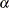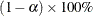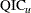### Displayed Output for Classical Analysis

The following output is produced by the GENMOD procedure. Note that some of the tables are optional and appear only in conjunction with the REPEATED statement and its options or with options in the MODEL statement. For details, see the section ODS Table Names.

#### Model Information

The Model Information table displays the two-level data set name, the response distribution, the link function, the response variable name, the offset variable name, the frequency variable name, the scale weight variable name, the number of observations used, the number of events if events/trials format is used for response, the number of trials if events/trials format is used for response, the sum of frequency weights, the number of missing values in data set, and the number of invalid observations (for example, negative or 0 response values with gamma distribution or number of observations with events greater than trials with binomial distribution).

#### Class Level Information

If you use classification variables in the model, PROC GENMOD displays the levels of classification variables specified in the CLASS statement and in the MODEL statement. The levels are displayed in the same sorted order used to generate columns in the design matrix.

#### Response Profile

If you specify an ordinal model for the multinomial distribution, a table titled Response Profile is displayed containing the ordered values of the response variable and the number of occurrences of the values used in the model.

#### Iteration History for Parameter Estimates

If you specify the ITPRINT model option, PROC GENMOD displays a table containing the following for each iteration in the Newton-Raphson procedure for model fitting: the iteration number, the ridge value, the log likelihood, and values of all parameters in the model.

#### Criteria for Assessing Goodness of Fit

In the Criteria for Assessing Goodness of Fit table, PROC GENMOD displays the degrees of freedom for deviance and Pearson’s chi-square, equal to the number of observations minus the number of regression parameters estimated, the deviance, the deviance divided by degrees of freedom, the scaled deviance, the scaled deviance divided by degrees of freedom, Pearson’s chi-square, Pearson’s chi-square divided by degrees of freedom, the scaled Pearson’s chi-square, the scaled Pearson’s chi-square divided by degrees of freedom, the log likelihood (excludes factorial terms) the full log likelihood, the Akaike information criterion, the corrected Akaike information criterion, and the Bayesian information criterion. The information in this table is valid only for maximum likelihood model fitting, and the table is not printed if the REPEATED statement is specified.

#### Last Evaluation of the Gradient

If you specify the model option ITPRINT, the GENMOD procedure displays the last evaluation of the gradient vector.

#### Last Evaluation of the Hessian

If you specify the model option ITPRINT, the GENMOD procedure displays the last evaluation of the Hessian matrix.

#### Analysis of (Initial) Parameter Estimates

The Analysis of (Initial) Parameter Estimates table contains the results from fitting a generalized linear model to the data. If you specify the REPEATED statement, these GLM parameter estimates are used as initial values for the GEE solution, and are displayed only if the PRINTMLE option in the REPEATED statement is specified. For each parameter in the model, PROC GENMOD displays the parameter name, as follows:

• the variable name for continuous regression variables

• the variable name and level for classification variables and interactions involving classification variables

• SCALE for the scale variable related to the dispersion parameter

In addition, PROC GENMOD displays the degrees of freedom for the parameter, the estimate value, the standard error, the Wald chi-square value, the p-value based on the chi-square distribution, and the confidence limits (Wald or profile likelihood) for parameters.

#### Lagrange Multiplier Statistics

If you specify that either the model intercept or the scale parameter is fixed, for those distributions that have a distribution scale parameter, the GENMOD procedure displays a table of Lagrange multiplier, or score, statistics for testing the validity of the constrained parameter that contains the test statistic, and the p-value.

#### Estimated Covariance Matrix

If you specify the model option COVB, the GENMOD procedure displays the estimated covariance matrix, defined as the inverse of the information matrix at the final iteration. This is based on the expected information matrix if the EXPECTED option is specified in the MODEL statement. Otherwise, it is based on the Hessian matrix used at the final iteration. This is, by default, the observed Hessian unless altered by the SCORING option in the MODEL statement.

#### Estimated Correlation Matrix

If you specify the CORRB model option, PROC GENMOD displays the estimated correlation matrix. This is based on the expected information matrix if the EXPECTED option is specified in the MODEL statement. Otherwise, it is based on the Hessian matrix used at the final iteration. This is, by default, the observed Hessian unless altered by the SCORING option in the MODEL statement.

#### Iteration History for LR Confidence Intervals

If you specify the ITPRINT and LRCI model options, PROC GENMOD displays an iteration history table for profile likelihood-based confidence intervals. For each parameter in the model, PROC GENMOD displays the parameter identification number, the iteration number, the log-likelihood value, parameter values.

#### Likelihood Ratio-Based Confidence Intervals for Parameters

If you specify the LRCI and the ITPRINT options in the MODEL statement, a table is displayed that summarizes profile likelihood-based confidence intervals for all parameters. For each parameter in the model, the table displays the confidence coefficient, the parameter identification number, lower and upper endpoints of confidence intervals for the parameter, and values of all other parameters at the solution.

#### LR Statistics for Type 1 Analysis

If you specify the TYPE1 model option, a table is displayed that contains the name of the effect, the deviance for the model including the effect and all previous effects, the degrees of freedom for the effect, the likelihood ratio statistic for testing the significance of the effect, and the p-value computed from the chi-square distribution with the effect’s degrees of freedom.

If you specify either the SCALE=DEVIANCE or SCALE=PEARSON option in the MODEL statement, columns are displayed that contain the name of the effect, the deviance for the model including the effect and all previous effects, the numerator degrees of freedom, the denominator degrees of freedom, the chi-square statistic for testing the significance of the effect, the p-value computed from the chi-square distribution with numerator degrees of freedom, the F statistic for testing the significance of the effect, and the p-value based on the F distribution.

#### Iteration History for Type 3 Contrasts

If you specify the model options ITPRINT and TYPE3, an iteration history table is displayed for fitting the model with Type 3 contrast constraints for each effect that contains the effect name, the iteration number, the ridge value, the log likelihood, and values of all parameters.

#### LR Statistics for Type 3 Analysis

If you specify the TYPE3 model option, a table is displayed that contains, for each effect in the model, the name of the effect, the likelihood ratio statistic for testing the significance of the effect, the degrees of freedom for the effect, and the p-value computed from the chi-square distribution.

If you specify either the SCALE=DEVIANCE or SCALE=PEARSON option in the MODEL statement, columns are displayed that contain the name of the effect, the likelihood ratio statistic for testing the significance of the effect, the F statistic for testing the significance of the effect, the numerator degrees of freedom, the denominator degrees of freedom, the p-value based on the F distribution, and the p-value computed from the chi-square distribution with the numerator’s degrees of freedom.

#### Wald Statistics for Type 3 Analysis

If you specify the TYPE3 and WALD model options, a table is displayed that contains the name of the effect, the degrees of freedom of the effect, the Wald statistic for testing the significance of the effect, and the p-value computed from the chi-square distribution.

#### Parameter Information

If you specify the ITPRINT, COVB, CORRB, WALDCI, or LRCI option in the MODEL statement, or if you specify a CONTRAST statement, a table is displayed that identifies parameters with numbers, rather than names, for use in tables and matrices where a compact identifier for parameters is helpful. For each parameter, the table contains an index number that identifies the parameter, and the parameter name, including level information for effects containing classification variables.

#### Observation Statistics

If you specify the OBSTATS option in the MODEL statement, PROC GENMOD displays a table containing miscellaneous statistics. Residuals and case deletion diagnostic statistics are not available for the multinomial distribution. Case deletion diagnostics are not available for zero-inflated models.

For each observation in the input data set, the following are displayed:

• the value of the response variable

• the predicted value of the mean

• the value of the linear predictor The value of an OFFSET variable is added to the linear predictor.

• the estimated standard error of the linear predictor

• the value of the negative of the weight in the Hessian matrix at the final iteration. This is the expected weight if the EXPECTED option is specified in the MODEL statement. Otherwise, it is the weight used in the final iteration. That is, it is the observed weight unless the SCORING= option has been specified.

• approximate lower and upper endpoints for a confidence interval for the predicted value of the mean

• raw residual

• Pearson residual

• deviance residual

• standardized Pearson residual

• standardized deviance residual

• likelihood residual

• Cook’s distance statistic

• DFBETA statistic, for each parameter

• standardized DFBETA statistic, for each parameter

• zero-inflation probability for zero-inflated models

• response mean for zero-inflated models

#### ESTIMATE Statement Results

If you specify a REPEATED statement, the ESTIMATE statement results apply to the specified GEE model. Otherwise, they apply to the specified generalized linear model.

For each ESTIMATE statement, the table contains the contrast label, the estimated value of the contrast, the standard error of the estimate, the significance level,confidence intervals for contrast, the Wald chi-square statistic for the contrast, and the p-value computed from the chi-square distribution. The mean of the contrast, defined as the inverse link function applied to the contrast, andconfidence intervals for the mean are also displayed.

If you specify the EXP option, an additional row is displayed with statistics for the exponentiated value of the contrast.

#### CONTRAST Coefficients

If you specify the CONTRAST or ESTIMATE statement and you specify the E option, a table titled Coefficients For Contrast label is displayed, where label is the label specified in the CONTRAST statement. The table contains the contrast label, and the rows of the contrast matrix.

#### Iteration History for Contrasts

If you specify the ITPRINT option, an iteration history table is displayed for fitting the model with contrast constraints for each effect. The table contains the contrast label, the iteration number, the ridge value, the log likelihood, and values of all parameters.

#### CONTRAST Statement Results

If you specify a REPEATED statement, the CONTRAST statement results apply to the specified GEE model. Otherwise, they apply to the specified generalized linear model.

A table is displayed that contains the contrast label, the degrees of freedom for the contrast, and the likelihood ratio, score, or Wald statistic for testing the significance of the contrast. Score statistics are used in GEE models, likelihood ratio statistics are used in generalized linear models, and Wald statistics are used in both. Also displayed are the p-value computed from the chi-square distribution, and the type of statistic computed for this contrast: Wald, LR, or score.

If you specify either the SCALE=DEVIANCE or SCALE=PEARSON option for generalized linear models, columns are displayed that contain the contrast label, the likelihood ratio statistic for testing the significance of the contrast, the F statistic for testing the significance of the contrast, the numerator degrees of freedom, the denominator degrees of freedom, the p-value based on the F distribution, and the p-value computed from the chi-square distribution with numerator degrees of freedom.

#### LSMEANS Coefficients

If you specify the LSMEANS statement and you specify the E option, the Coefficients for effect Least Squares Means table is displayed, where effect is the effect specified in the LSMEANS statement. The table contains the effect names and the rows of least squares means coefficients.

#### Least Squares Means

If you specify the LSMEANS statement, the Least Squares Means table is displayed. The table contains for each effect the following: the effect name, and for each level of each effect the following:

• the least squares mean estimate

• standard error

• chi-square value

• p-value computed from the chi-square distribution

If you specify the DIFF option, a table titled Differences of Least Squares Means is displayed containing corresponding statistics for the differences between the least squares means for the levels of each effect.

#### GEE Model Information

If you specify the REPEATED statement, the GEE Model Information table displays the correlation structure of the working correlation matrix or the log odds ratio structure, the within-subject effect, the subject effect, the number of clusters, the correlation matrix dimension, and the minimum and maximum cluster size.

#### Log Odds Ratio Parameter Information

If you specify the REPEATED statement and specify a log odds ratio model for binary data with the LOGOR= option, then the Log Odds Ratio Parameter Information table is displayed showing the correspondence between data pairs and log odds ratio model parameters.

#### Iteration History for GEE Parameter Estimates

If you specify the REPEATED statement and the MODEL statement option ITPRINT, the Iteration History For GEE Parameter Estimates table is displayed. The table contains the parameter identification number, the iteration number, and values of all parameters.

#### Last Evaluation of the Generalized Gradient and Hessian

If you specify the REPEATED statement and select ITPRINT as a model option, PROC GENMOD displays the Last Evaluation Of The Generalized Gradient And Hessian table.

#### GEE Parameter Estimate Covariance Matrices

If you specify the REPEATED statement and the COVB option, PROC GENMOD displays the Covariance Matrix (Model-Based) and Covariance Matrix (Empirical) tables.

#### GEE Parameter Estimate Correlation Matrices

If you specify the REPEATED statement and the CORRB option, PROC GENMOD displays the Correlation Matrix (Model-Based) and Correlation Matrix (Empirical) tables.

#### GEE Working Correlation Matrix

If you specify the REPEATED statement and the CORRW option, PROC GENMOD displays the Working Correlation Matrix table.

#### GEE Fit Criteria

If you specify the REPEATED statement, PROC GENMOD displays the quasi-likelihood information criteria for model fit QIC andin the GEE Fit Criteria table.

#### Analysis of GEE Parameter Estimates

If you specify the REPEATED statement, PROC GENMOD uses empirical standard error estimates to compute and display the Analysis Of GEE Parameter Estimates Empirical Standard Error Estimates table that contains the parameter names as follows:

• the variable name for continuous regression variables

• the variable name and level for classification variables and interactions involving classification variables

• Scale for the scale variable related to the dispersion parameter

In addition, the parameter estimate, the empirical standard error, a 95% confidence interval, and the Z score and p-value are displayed for each parameter.

If you specify the MODELSE option in the REPEATED statement, the Analysis Of GEE Parameter Estimates Model-Based Standard Error Estimates table based on model-based standard errors is also produced.

#### GEE Observation Statistics

If you specify the OBSTATS option in the REPEATED statement, PROC GENMOD displays a table containing miscellaneous statistics. For each observation in the input data set, the following are displayed: